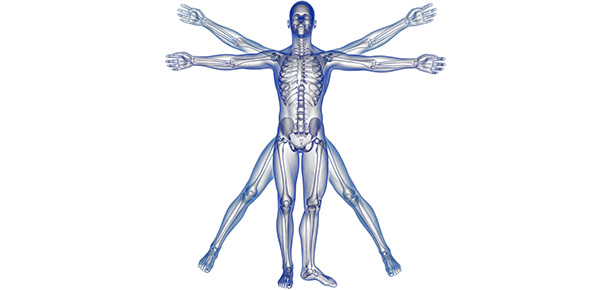# Physiology Calculations Walkthrough

9 Questions | Total Attempts: 55SettingsAll about essential renal physiology calculations from eGFR, to RPF and everything in between. Also, watch out for the question especially relating to our scenario regarding diabetes nephropathy as well as a revision question for the last session about Na and K balance. :)

Related Topics
• 1.
Using these results:Urine Flow Rate: 1ml/minPlasma Concentration of Inulin: 100mg/mlUrine Concentration of Inulin: 12g/mlThe value for eGFR is _______ ml/min.
• 2.
The ratio of extracellular to intracellular compartment volume is:
• A.

2/3:1/3

• B.

1:3

• C.

1:2

• D.

3:1

• 3.
In order to achieve a more accurate measure of glomerular filtration rate is utilising SNGFR which is calculated through the equation Kf { ( P[GC] - P[T] ) - ( π[GC] - π[T] ) }. This is basically the Kf (Net _________ Pressure - Net Colloid Osmotic Pressure).
• 4.
Is the following statement true or false: the transport maximum of a substance = GFR x ideal renal threshold of that substance.
• A.

True

• B.

False

• 5.
Using these results:Urine Flow Rate: 1ml/minPlasma Concentration of A: 10mg/mlUrine Concentration of A: 2g/mlWhat is the rate of reabsorption or secretion for Substance A (assuming it is freely filtered)? (Don't forget units!)
• 6.
What is the meaning of the filtration fraction (why does it even exist) ? As a bonus, what's the normal value of FF?
• 7.
Tick the correct response/s regarding acid-base balance:
• A.

New bicarbonate generated = Net Acid Excretion = ( titratable acid - ammonium - bicarbonate )

• B.

A symptom of person with uncontrolled diabetes mellitus is a urinary pH below 5.

• C.

Retaining bicarbonate is equivalent to absorbing a proton.

• D.

If the pH is known, the Henderson-Hasselbalch equation can be used to calculate the relative concentrations of Ha and A-.

• 8.
Is an increased or decreased Na content in the urine a symptom of diabetes nephropathy?
• A.

Decreased

• B.

Increased